# The changing impact of parenthood on earnings inequality

In this project, I develop a method to estimate the impact of parenthood on earnings inequality as measured with the squared coefficient of variation. Since the effect of parenthood on earnings inequality depends on the households’ position in the earnings distribution, I decompose the squared coefficient of variation into additive subgroupsthat represent low-, medium-, and high-income households, respectively.

The between-group component of the squared coefficient of variation then is

(1)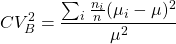(2)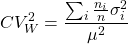The within-group component of the squared coefficient of variation is:

In order to understand the impact of parenthood on those statistics, I take the derivative with respect to childbirth:

(3)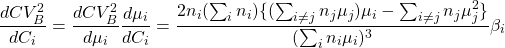(4)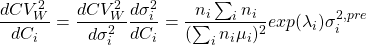The group sizes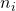, the mean earnings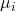can directly be retrieved from data. The effect of childbirth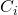on mean earnings,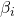, and the effect of childbirth on the variance of earnings,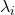, can be modeled using linear regression and variance function regression, respectively.

I am using this approach currently to understand the changing impact of parenthood on earnings inequality from 1990 to 2020.